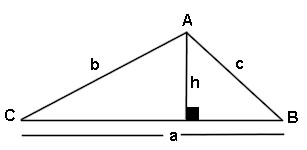# Triangle Area Side Side Angle Calculator

This calculator requires the use of Javascript enabled and capable browsers. This calculator is designed to give the area of a triangle, assuming two sides and an angle not between the sides are known, also known as SSA. There are three possible situations and solutions, since it may not be possible to calculate a unique triangle; no triangle, one triangle or two triangles. Enter the two known sides and the angle. Then click on calculate. In a triangle, all interior angles total to 180 degrees. No two angles can total to 180 degrees or more. It is for any shape triangle. Click on Calculate. Any of the three conditions may be returned. In case you need them, here are the Trig Triangle Formula Tables, as well as the Right Triangle Angle And Side Calculator. Here is our Triangle Math Menu.

 Unit Number Required Data Entry Quantity Decimal Places Angle Units Designation degrees (decimal) radians Angle A Not Between Known Sides a & b Angle Units Known Side a Length Units Known Side b Length Units Calculated Results Triangle 1 Calculated Angle B Angle Units Calculated Angle C Angle Units Calculated Side c Length Units Area Of Triangle Square Units Calculated Results Triangle 2 Calculated Angle B Angle Units Calculated Angle C Angle Units Calculated Side c Length Units Area Of Triangle Square UnitsUpdated 8.15.11

 Leave us a question or comment on FacebookSearch or Browse Our Site